# What are Equivalent Fractions?

Equivalent fractions are  fractions which has the same value although they look different from each other.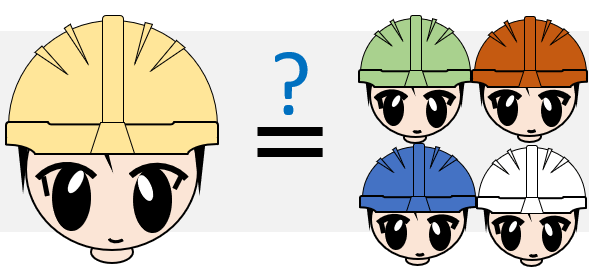I will be explaining equivalent fractions in layman’s term as you move forward in this lesson.

For those who do not understand fractions yet, please visit my post entitled “What is a Fraction?”. Your basic knowledge about fractions will help you understand this topic easily.

In this lesson, we will learn what is equivalent fractions and how to find them.

### Why do we need to know or learn equivalent fractions?What is the importance of equivalent fractions? We need to learn equivalent fraction for the following important reasons:

1. Knowledge in equivalent fraction will help you to understand simplifying fractions easily.

2. Knowledge in equivalent fraction is required in addition and subtraction of fractions (particularly improper fractions).

### What is an equivalent fraction?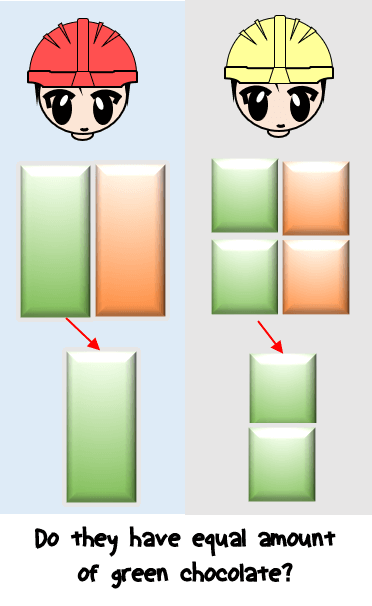In our introduction, equivalent fractions are fractions which has same value although they look different from each other.

It means that these fractions are equal.

However, they look different from each.

In this definition, we are talking about two things: (1) their appearance, and (2) their value.When we say different from each other, it means that the numerator of one fraction is not the same number with the numerator of the other fraction.

Similarly, their denominators are not same number as well.

But although with those differences, they have equal value.

This is what we mean for equivalent fraction.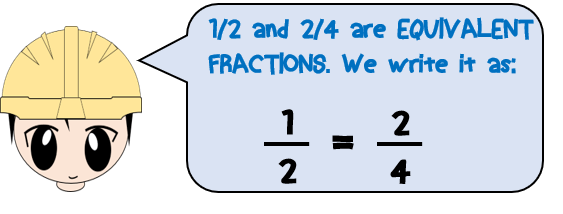### Examples of equivalent fractions.

To understand equivalent fractions better, let us have some examples.

1/2, 2/4, 3/6, 4/8, and 5/10 are example of equivalent fractions. They are not necessarily in that order.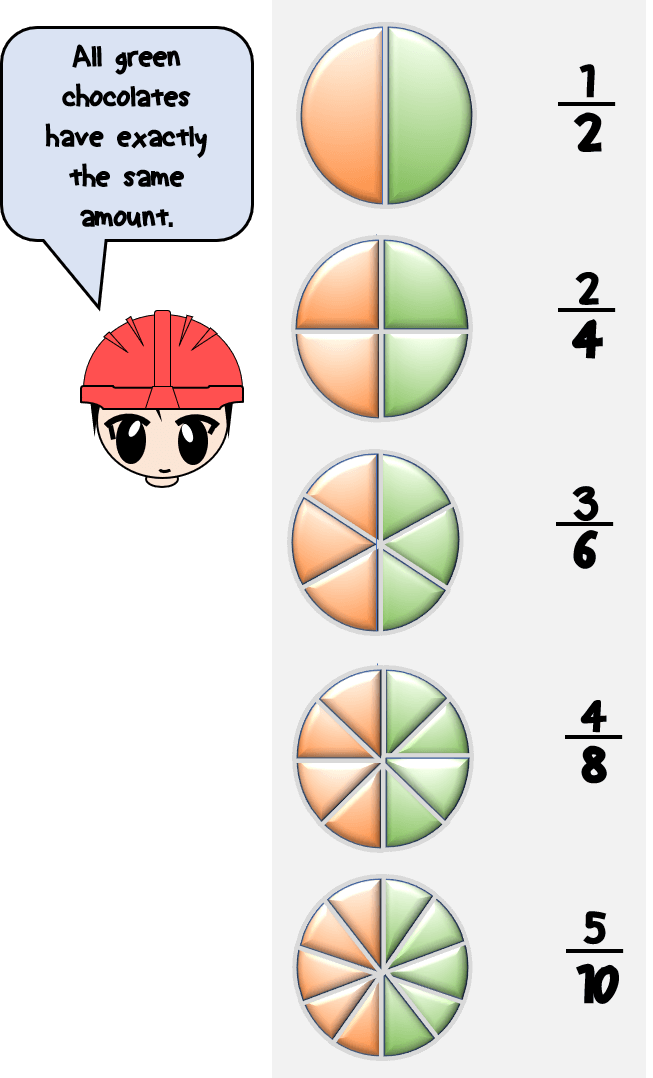It means that 1/2 is equal to 2/4, 3/6, 4/8 and 5/10.

1/2 is the lowest equivalent fraction out of 5 because it has the smallest numerator and denominator. It cannot be also reduced any further. But please remember that although 1/2 has the smallest numerator and denominator, it has the same value with its equivalent fractions 2/4, 3/6, 4/8, and 5/10.

Similarly, 4/8 is equal to 1/2, 2/4, 3/6, and 5/10.

Do not be confused by the order or arrangement.Above are just only 5 examples of equivalent fractions. But actually, you can draw an infinite numbers of equivalent fractions for 1/2, 2/4, 3/6, 4/8, and 5/10. If we will continue, they are equivalent also with 6/12, 7/14, 8/16, 9/18, 10/20 and so on.

Let us have another example.

The equivalent fraction for 1/3 is 2/6, 3/9, 4/12, and so on.

Can you find other equivalent fractions of 1/3?

### Steps to Find Equivalent FractionTo obtain any equivalent fractions, we need to follow 2 simple steps below:

STEP 1. Think of any whole number. This number will be used to find equivalent fraction of our given fraction.

STEP 2. Multiply or divide the numerator and the denominator of our fraction with the whole number we obtained in step 1.

The result after performing step 2 is our equivalent fraction.

Let us analyze our steps. If you already understand the steps above, you can proceed directly to our examples.

STEP 1. Think of any whole number except 1. This number will be used to find equivalent fraction of our given fraction.

What does “whole number except 1” mean?

Whole number except 1 means any of the counting numbers (except 1) like 2, 3, 4, 5, 6, 7, and so on. There are infinite numbers that can be used to find equivalent fraction of any specific fraction.

STEP 2. Multiply or divide the numerator and the denominator of our fraction with the whole number we obtained in step 1.

How to do the multiplication or division as what step 2 describe

a. Finding equivalent fraction by MULTIPLICATION:

To find equivalent fraction by multiplication, multiply the numerator and the denominator with the same whole number we have selected in step 1. The result after performing the multiplication will be our equivalent fraction. We can come up with infite number of equivalent fraction using multiplication technique.  See example below.b. Finding equivalent fraction by DIVISION:

To find equivalent fraction by division, divide the numerator and the denominator with the same whole number we have selected in step 1.  The result after performing the division will be our equivalent fraction.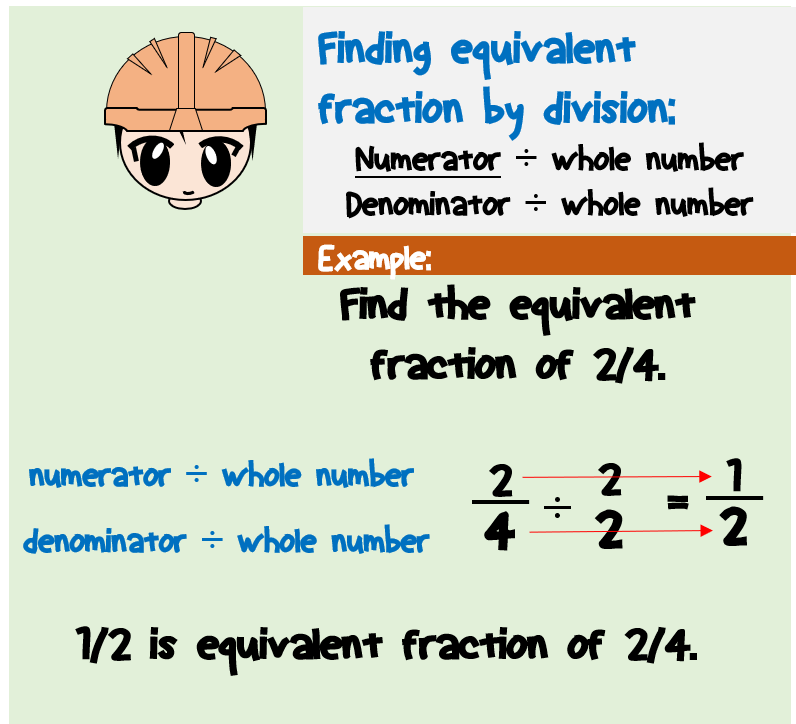However, we cannot just select any whole number when finding equivalent fraction by division. We need to select a whole number that can be divided to our numerator and our denominator without any remainder. We can come up with the simplified equivalent fraction when doing this continuously.  See example below.

Why has it to be multiplied or divide? Why is it not to multiply only? Or why is it not to divide only?

We will not be performing multiplication or division at the same time. Depending upon the problem, we will choose either to multiply or to divide.

If we need to find equivalent fraction which is greater than our original fraction, we need to multiply.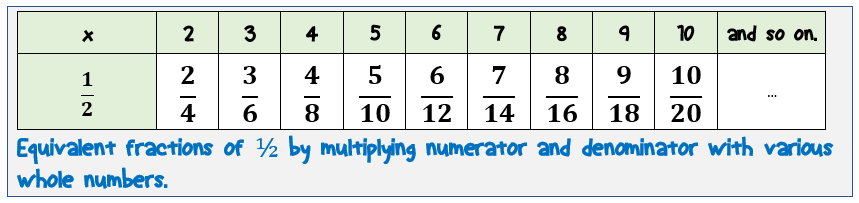If we need to find equivalent fraction which is lesser than our original fraction, we need to divide.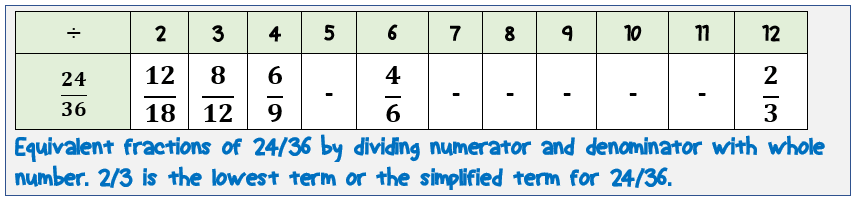We know that equivalent fractions have the same value. Why do the value of our original fraction are not changing after doing the multiplication or the division as mentioned in step 2?

The value of our original fraction are not changing after doing the multiplication and division because it is like we multiply or divide our fraction by a value equal to one. Please note that any number multiplied or divided by one will not change the value.

### Do Equivalent Fractions – Examples

We will understand above steps better by looking at some examples below. We will be solving three different problems which will cover all possible questions related to equivalent fractions.

### Find at least two equivalent fractions of 1/2.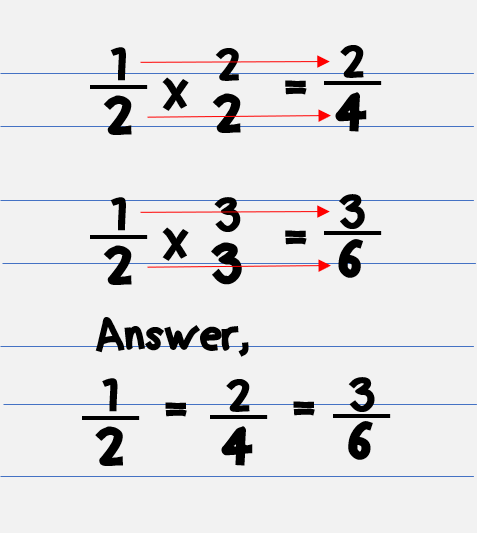Step 1. “Think of any whole number except 1. This number will be used to find equivalent fraction of our given fraction.”

In this case, we are asked to find any but at least two equivalent fractions.

I will be using 2 and 3 as my whole number.

I can use also other whole numbers such as 4, 5, 6, and so on. But for now, I will be using 2 and 3 because small numbers are easy to calculate.

Now that I have my whole numbers, let us proceed to step 2.

Step 2. Multiply or divide the numerator and the denominator of our fraction with the whole number we obtained in step 1

Multiply what we have obtained in step 1 to our fraction 1/2.

It means that I will multiply 2 to our numerator and denominator.  The result will give us 2/4. This is our first equivalent fraction.

I will do the same for the second equivalent fraction. I will multiply 3 to the numerator and the denominator.  It will give us 3/6.

Thus, 2/4 and 3/6 are some of the equivalent fractions.

Note: You can have different results if you use other whole numbers in step 1 and it will still be correct.

### Find the equivalent fractions of 2/3 with denominator equal to 12.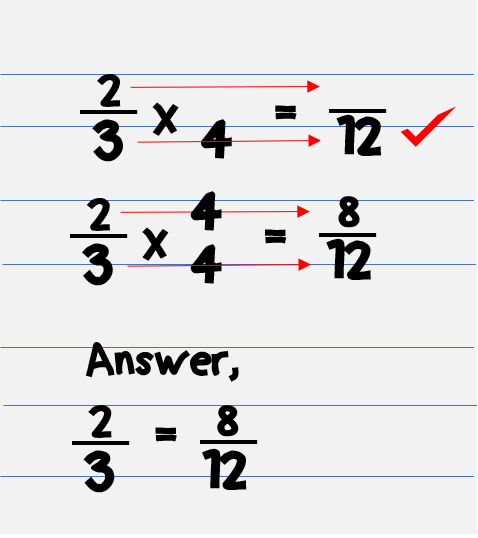Step 1.

In this problem, we are asked to find an equivalent fractions of 2/3 but the denominator must be equal to 12.

The clue in this problem is the denominator which must be equal to 12.

Now, in order for 2/3 to have an equivalent fraction with denominator equal to 12, we have to multiply something to it.

I will think of a whole number that will result a denominator equal to 12 when multiplied to our denominator 3.

If we will use 4, it seems that it will give what we are looking for?

Thus, let us select 4.

Step 2.

Multiply 4 to the numerator and the denominator of 2/3.

The result will give us 8/12. The denominator is equal to 12 which exactly what we are looking for.

Thus, the equivalent of 2/3 with denominator equal to 12 is 8/12.

### What is the lowest term of a fraction 8/10?

The lowest term of a fraction means:

1. An equivalent fraction with the lowest numerator and denominator.
2. Cannot be divided any further without having a remainder.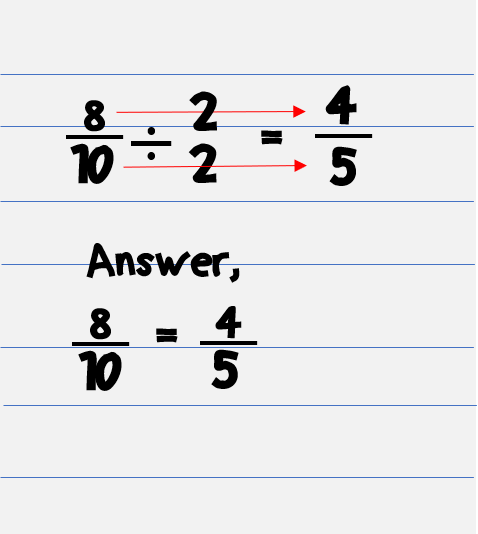Step 1.

In this problem, we are asked to find an equivalent fraction of 8/10 with the lowest numerator and denominator.

It means that we have to divide something to 8/10.

If I will select 4, it can be divided to our numerator 8 without a remainder. However, if will divide it to our denominator it will have a remainder. Thus, 4 cannot be used as our whole number in this problem.

Let us select 2 because 2 can be divided to our numerator and denominator without any remainder.

Proceed to step 2.

Step 2.

Divide the numerator and the denominator of our fraction with 2.

The result will be 4/5.

Is their any whole number that can be divided to both numerator and denominator without any remainder? None.

Thus, the answer is 4/5.

Note: If ever, the resulting equivalent fraction can still be divided further to make it lower, then just repeat step 1 and step 2.

### Related Topics

1. What is a Fraction?

2. What are Equivalent Fractions?